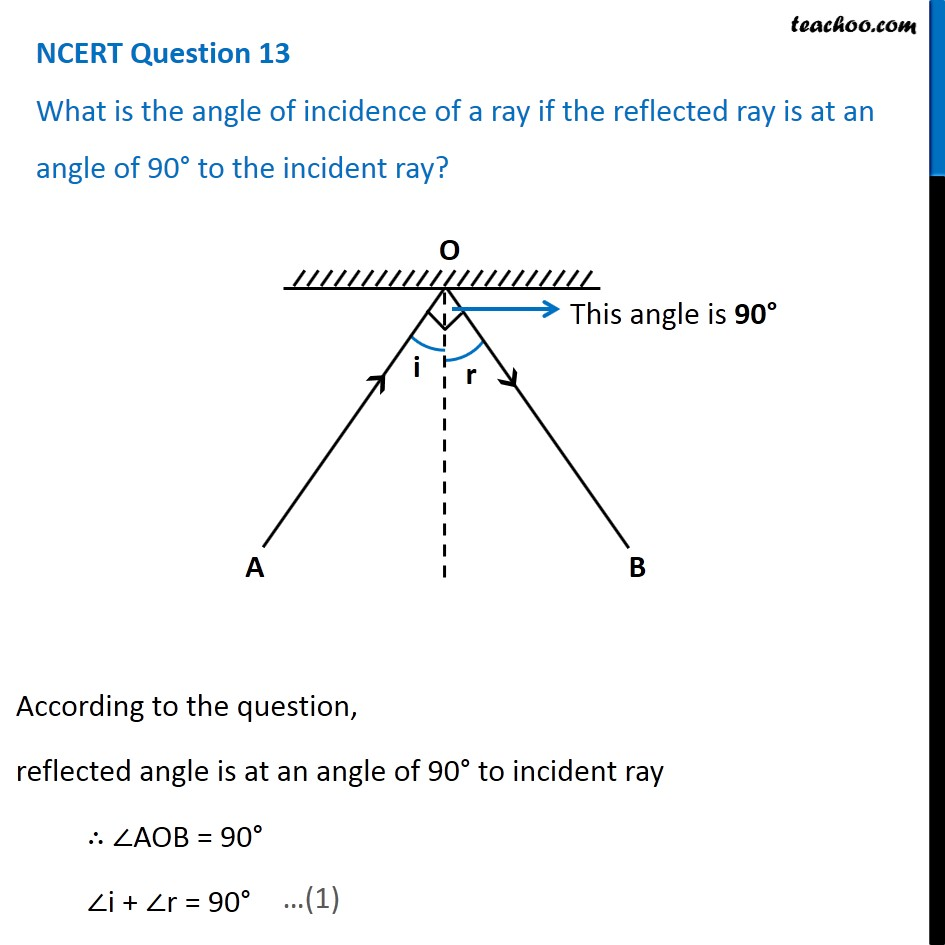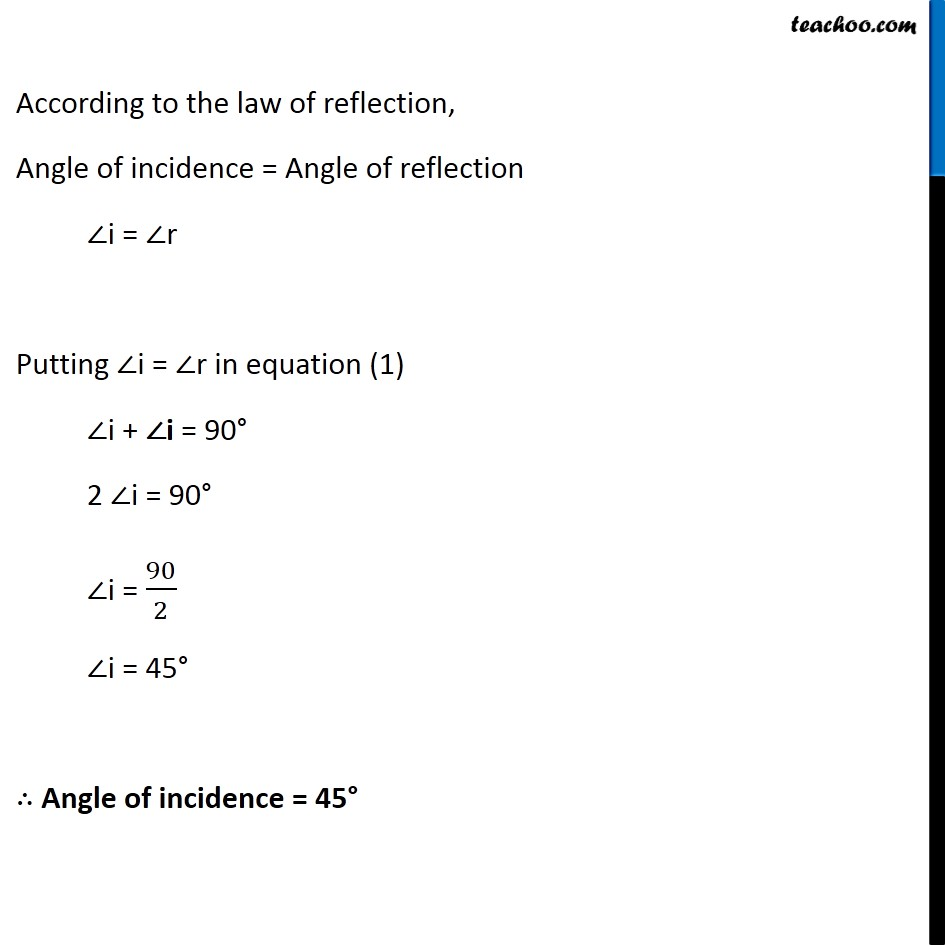## What is the angle of incidence of a ray if the reflected ray is at an angle of 90° to the incident ray?

###1. Class 8
2. Chapter 16 Class 8 - Light
3. NCERT Questions

Transcript

According to the question, reflected angle is at an angle of 90° to incident ray ∴ ∠AOB = 90° ∠i + ∠r = 90° …(1) According to the law of reflection, Angle of incidence = Angle of reflection ∠i = ∠r Putting ∠i = ∠r in equation (1) ∠i + ∠i = 90° 2 ∠i = 90° ∠i = 90/2 ∠i = 45° ∴ Angle of incidence = 45°

NCERT Questions

Class 8
Chapter 16 Class 8 - Light